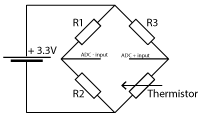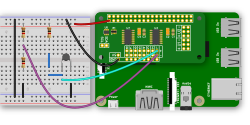# Knowledge Base## ADC Differential Pi with NTC Thermistors

### Using an ADC Differential Pi with NTC ThermistorsCreated: 30/10/2015 | Last Updated: 22/05/2021

In this tutorial we will use the ADC Differential Pi or DeltaSigma Pi with a Semitec103AT-11 NTC (Negative Temperature Coefficient) Thermistors 10kohm 1% to make a digital thermometer. For this you will need your Raspberry Pi, an ADC Differential Pi or DeltaSigma Pi, and a Semitec103AT-11 NTC.

We will use the AB Electronics python library to talk to the ADC Differential Pi, to download the library visit our Python Library and Demos knowledge base article.

You will need to enable i2c on your Raspberry Pi, see our other tutorial on i2c: I2C, SMBus and Raspbian Linux

## Parts Used:

Semitec103AT-11 NTC (Datasheet)

3 x 10K Resistor

Connecting Wire

## Connecting the Sensor and resistors to the ADC Differential Pi PlusIf you haven’t done so install your ADC Differential Pi Plus onto the Raspberry Pi by connecting it onto the GPIO header.  Make sure your Raspberry Pi is turned off when you do this to minimise the risk of damaging the Raspberry Pi or the ADC Differential Pi Plus.

This circuit uses a "Wheatstone bridge" to return a voltage dependent on the resistance of the temperature sensor and using this we can then calculate the resistance and then the temperature.

On a breadboard add the 3 x 10K resistors and the temperature sensor as shown on the circuit mock up image (you can click to enlarge)

Connect the ADC + input from the breadboard to the + channel on input 1 on the Differential Pi board.

Connect the ADC - input from the breadboard to the - channel on input 1 on the Differential Pi board.On the ADC Differential Pi connect the negative inputs on channels 1, 2 and 3 to GND, this sets the ADC chips into single ended mode to measure between 0 and 2.048 volts.

For this tutorial we will start by creating a new python program file called demo-demo-resistance-thermometer.py.  You can use your favourite text editor for writing the program.  You can find a complete example of demo_resistance_thermometer.py in the ABElectronics_Python_Libraries/ADCDifferentialPi/demos/ folder.

At the top of your program you will need to import the ADCDifferentialPi library and time library. We also add variables to hold the values related to the sensor data conversion.

```#!/usr/bin/env python
import time
import os
import math
```

The ADCDifferentialPi library is used for all communication with your ADC Differential Pi Plus, it gives you control over almost everything that can be done with the MCP3424 controller.

Next we need to create an instance of the ADCDifferentialPi class and call it adc

```adc = ADCDifferentialPi(0x68, 0x69, 18)
```

0x68 and 0x69 are the I2C address for the ADC chips, if you have changed the address selection jumpers on your ADC Differential Pi Plus then you will need to change these numbers to match the new addresses.

Now we add variables which will be used to hold the default values of the resistors and thermometer values from the datasheet.

```# the resistor values for the Wheatstone bridge are:
resistor1 = 10000
resistor2 = 10000
resistor3 = 10000
# Input voltage
voltin = 3.3
# Resistance thermometer values from datasheet
bResistance = 3435
t25Resistance = 10000
# Constants
t0 = 273.15;
t25 = t0 + 25;
```

We need to create new function to convert the voltage returned into the resistance using:

```def calcResistance(voltage):
return (resistor2*resistor3 + resistor3* (resistor1+resistor2)*voltage / voltin )/ (resistor1- (resistor1+resistor2)*voltage / voltin)
```

We need to create new function to convert the resistance into the temperature:

```def calcTemp(resistance):
return 1 / ( (math.log(resistance / t25Resistance) / bResistance) + (1 / t25) ) - t0;
```

We are going to need is a loop so that the same commands can run over and over.  This can be done with a simple while loop.

```while True:
```

As True is always true the while loop will keep running until you exit the program with a Ctrl-C.

Now we read the voltage from the ADC channel and convert to the values needed:

```# read from adc channels and print to screen
thermresistance = calcResistance(bridgeVoltage)
temperature = calcTemp(thermresistance)
```

In order to clear the console for each read we need to issue a clear command.

```# clear the console
os.system('clear')
```

Next we print the values to the screen.

```# print values to screen
print("Bridge Voltage: %02f volts" % bridgeVoltage)
print("Resistance: %d ohms" % thermresistance)
print("Temperature: %.2fC" % temperature)
```

Using the time.sleep method we add a 0.5 second delay before repeating the read again.

```time.sleep(0.5)
```

time.sleep takes one variable, a number which represents the number of seconds to wait.  1 will make the program sleep for 1 second while 0.1 would wait for 100ms.

That is everything we need to make read from the Thermistor sensor using your ADC Differential Pi or Delta Sigma Pi, your program should now look like this.

```#!/usr/bin/env python
import time
import os
import math

"""
================================================
using a Wheatstone bridge.
This demo uses a Semitec NTC (Negative Temperature Coefficient) Thermistors
10kohm 1%, Manufacturer Part No: 103AT-11
Purchased from Mouser Electronics, Part No: 954-103AT-11
The circuit is connected to the + and - inputs on channel 7 on the
ADC Differential Pi. This can also be used on the Delta Sigma Pi
The Wheatstone bridge is comprised of three 10K resistors and the
Resistance thermometer
Version 1.0 Created 30/10/2015
Requires python3 smbus to be installed
run with: python3 demo_resistance_thermometer.py
================================================
Initialise the ADC device using the default addresses and 18 bit sample rate,
change this value if you have changed the address selection jumpers
Bit rate can be 12,14, 16 or 18
"""

# the resistor values for the Wheatstone bridge are:
resistor1 = 10000
resistor2 = 10000
resistor3 = 10000

# Input voltage
voltin = 3.3

# Resistance thermometer values from datasheet
bResistance = 3435
t25Resistance = 10000

# Constants
t0 = 273.15;
t25 = t0 + 25;

def calcResistance(voltage):
return (resistor2*resistor3 + resistor3* (resistor1+resistor2)*voltage / voltin )/ (resistor1- (resistor1+resistor2)*voltage / voltin)

def calcTemp(resistance):
return 1 / ( (math.log(resistance / t25Resistance) / bResistance) + (1 / t25) ) - t0;

# loop forever reading the values and printing them to screen
while (True):
thermresistance = calcResistance(bridgeVoltage)
temperature = calcTemp(thermresistance)

# clear the console
os.system('clear')

# print values to screen
print("Bridge Voltage: %02f volts" % bridgeVoltage)
print("Resistance: %d ohms" % thermresistance)
print("Temperature: %.2fC" % temperature)

# wait 0.5 seconds before reading the pins again
time.sleep(0.5)
```

Save your program as "demo_resistance_thermometer.py" and run it in a command terminal using

```python demo_resistance_thermometer.py
```

You will now have reading from your sensor on the console which will repeat every 0.5 seconds.

(images created with Fritzing)